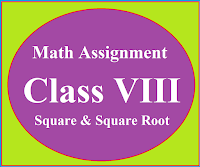### Math Assignment Class VIII | Square & Square RootMath Assignment  Class VIII | Square & Square Root Download or Print free  assignment with answer key  for   Class  8 Squares and  Square Roots.   Important and extra questions that cover all topics of square and square root and is useful and helpful for the students. Math Assignment  Class VIII | Square & Square Root LEVEL -1

## Maths Assignment  Class 9th Chapter 1

Math Assignment for Class IX Ch -1, Number System strictly according to the CBSE syllabus. Extra questions based on the topic Number System.

## MATHEMATICS ASSIGNMENT CLASS IX NUMBER SYSTEM

Q1- Insert 5 rational and 5 irrational numbers between
(a) 7 & 8,  (b) 2 & 3.2,   (c) 2.7 & 6.32,

Q2- Represent the following on the number line.

Q3- Express the following in p/q form

Q 4:  Evaluate

(a) 1⁸ × 3⁰ × 5³ × 2²           Ans; 500,

(b)  4-3 × 4⁸ ÷ 4²                Ans; 64

(c) 3⁷ ÷ 3⁴ × 3-6                 Ans

(d) 3-4 × 3-5 ÷ 3¹⁰             Ans; 3-19

Q5- Evaluate

Q6.  If    , then find the value of the followings

Q7.  Rationalize the denominator of

Q 8.  Rationalize the following and find the value of a and b

Q 9.  Simplify the followings

Q10  Show that 0.142857142857….=

Q11- Locate

on the number line.

Q 12.  Find the value of a and b  if

Q 13. Show that :

Q 14.  If x = , y =     find x²+y².   [ans; 98]

Q 15 Find the value of a and b if

Q 16. Simplify:      [ans; 0]

Q 17. Simplify:      [ans; 1]

Q 18.  Rationalize the denominator of

Q 19.

Q 20.  If     = 3.162, then find the value of        [Ans; 0.3162]
Q 21.  If 27ˣ =  then find x.        [Ans; 1/2]
Q 22. Simplify [5(81/3+271/3)³]1/4 .          [Ans; 5]
Q 23. Simplify- (1³ + 2³ + 3³)1/2.           [Ans; 6]

Q 24. If (5x-3)(32x-8) = 225 find x.    [Ans; 5]

Q 25.
Prove that :

🙏

1.Plese send us solution today is our test

2.Need solutions toooo